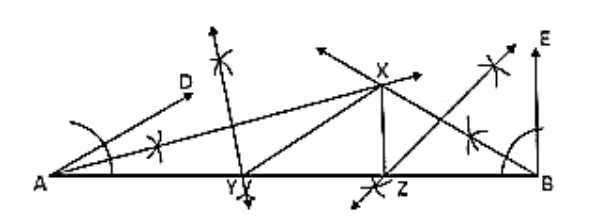# Construct a triangle XYZ in which ∠Y = 30°,

Question:

Construct a triangle XYZ in which ∠Y = 30°, ∠Z = 90° and XY + YZ + ZX = 11 cm.

Solution:Steps of construction:

1. Draw a line segment AB of 11 cm.

2. Draw ∠DAB = 30° and ∠FBA = 90°.

3. Draw the angle bisectors of ∠DAB and ∠EBA which intersect each other at X.

4. Draw the perpendicular bisector of XA and XB which intersect AB at Y and Z respectively

5. Join XY and XZ.

Hence △XYZ is the required triangle.